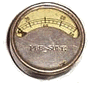# Geo Coordinate

This example illustrates the framework's accomodation of WholeValue(s). Geocoordinates were first introduced to Fit in the incomplete NetworkExample.

A geocoordinate is a coordinate used in geography (geo = geography). A coordinate is a pair of ordinates (co = pair). An ordinate is a measure of position (ordinate = ordinal number). Informally, a geocoordinate measures a position in the world.

A geocoordinate is the point where a vector from the center of the earth (for example) intersects the surface. That is, for a given surface, a two dimensional vector defines a three dimensional point in space.

Here we use the matematical notion of theta and phi to define a point and then give its cooresponding GeoCoordinate as lattitude and longitude north or south of the equator (phi=90) and east or west of the prime merridian (theta=0).

In this test we will find it convenient to specify ideal points (using degrees) and find their geocoordinates. Note, theta and phi are concepts used for writing this test, not necessarily properties of a geocoordinate.

 eg.GeoCoordinateExample theta phi coord() 10 60 10 e 30 n 350 120 10 w 30 s

We can also specify a GeoCoordinate and find the vector that represents it. When we do so we will expect 0 <= theta < 360 and 0 <= phi <=180, though that is just a convention of this test. We assume theta and phi are checked to the precision implied by the number of significant digits written.

 eg.GeoCoordinateExample coord theta() phi() 10 e 30 n 10 60 10 w 30 s 350 120

We accept some variety of notation. In general, a latitude or longitude can be specified with one, two or three numbers. These are consistently prefixed or sufixed with n, s, e, or w, possibly capitalized, spelt out, or omitted when signed numbers are assumed. Spaces and other punctuation is ignored except that it serves as a separator.

 eg.GeoCoordinateExample coord theta() phi() 10 30 10 60 -10 -30 350 70 10 east 30 north 10 60 10.5 e 30.5 n 10.5 59.5 10.5e, 30.5n 10.5 59.5 10.5e30n 10.5 60 10.5 E 30.5 N 10.5 59.5 e 10.5 n 30.5 10.5 60.5 10 30 e 30 30 n 10.5 59.5 10:30 e 30:30 n 10.5 59.5 10 30 00 e 30 30 00 n 10.5 59.5 10 30.5 e 30 30.5 n 10.508333 49.491666 10° 30' 30" e 30° 30' 30" n 10.508333 49.491666

We don't expect to encounter a lat or lon like the following so we don't specify behavior for the following cases other than to say: all possible inputs produce either a well formed geocoordinate, answer null or throw an exception.

• -100 w (for east?)
• 100 -5 (for 5 min west of 100 east?)

We depend on geocoordinates being equal when they specifiy the same point, even when they aren't specified the same way. Also, points that are very close are considered equal. We defined the boundary between very close (equal) and not as close (unequal) to be somewhere between 1/2 and 1/10 of a second of arc, measured independently in both latitude and longitude. This measure of closeness is overly stringent at the poles which is one more thing that is wrong there besides being very cold.

 eg.GeoCoordinateExample coord coord2 equal() 45.5 n 122.5 w N 45 30 00, W 122 30 00 true E 10 10 10, S 10 10 10.1 E 10 10 10, S 10 10 10.9 false E 10 10 10, S 10 10 10.11 E 10 10 10, S 10 10 10.19 true E 10 10 10, S 10 10 10.111 E 10 10 10, S 10 10 10.119 true

A classing example of using geocoordinates is that of finding the great-circle distance between places on the earth. This, of course, requires factoring in the three-dimensional nature of the path, the radius of the earth, and for good accuracy, the variation in radius due to the equatorial bulging (oblateness) of the earth's average surface. Here we compare distances (miles) computed using the WGS84 conventions to those assuming a spherical earth.

 eg.GeoCoordinateExample coord coord2 wgs84() sphere() route 45°35'19"N 122°35'51"W 22°18'32"N 113°54'53"E 6571.07 6555.10 portland to hong kong 47°26'56"N 122°18'34"W 33°56'46"S 151°10'38"E 7739.45 7744.53 seattle to sydney 61°10'28"N 149°59'47"W 51°28'39"N 00°27'41"W 4488.67 4470.68 ancorage to london

These are web resources that were used to produce some of these expected values.

Hint: A reasonable implementation might separate geocoordinates from the surface models that interpret them as points in space. There are actually quite a number of the latter as it turns out. Real topographic data could serve as a model also.

Last edited July 12, 2005